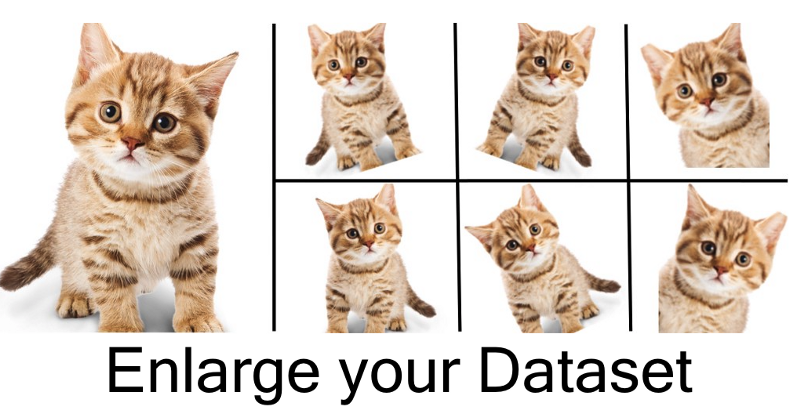Ford车（A品牌）却朝向右侧

## 4.1 翻转（Flip）

``````# NumPy.'img' = A single image.
flip_1 = np.fliplr(img)
# TensorFlow. 'x' = A placeholder for an image.
shape = [height, width, channels]
x = tf.placeholder(dtype = tf.float32, shape = shape)
flip_2 = tf.image.flip_up_down(x)
flip_3 = tf.image.flip_left_right(x)
flip_4 = tf.image.random_flip_up_down(x)
flip_5 = tf.image.random_flip_left_right(x)``````

## 4.2 旋转（Rotation）

``````# Placeholders: 'x' = A single image, 'y' = A batch of images
# 'k' denotes the number of 90 degree anticlockwise rotations
shape = [height, width, channels]
x = tf.placeholder(dtype = tf.float32, shape = shape)
rot_90 = tf.image.rot90(img, k=1)
rot_180 = tf.image.rot90(img, k=2)
# To rotate in any angle. In the example below, 'angles' is in radians
shape = [batch, height, width, 3]
y = tf.placeholder(dtype = tf.float32, shape = shape)
rot_tf_180 = tf.contrib.image.rotate(y, angles=3.1415)
# Scikit-Image. 'angle' = Degrees. 'img' = Input Image
# For details about 'mode', checkout the interpolation section below.
rot = skimage.transform.rotate(img, angle=45, mode='reflect')
``````

## 4.3 缩放比例（Scale）

``````# Scikit Image. 'img' = Input Image, 'scale' = Scale factor
# For details about 'mode', checkout the interpolation section below.
scale_out = skimage.transform.rescale(img, scale=2.0, mode='constant')
scale_in = skimage.transform.rescale(img, scale=0.5, mode='constant')
# Don't forget to crop the images back to the original size (for
# scale_out)
``````

## 4.4 裁剪（Crop）

``````# TensorFlow. 'x' = A placeholder for an image.
original_size = [height, width, channels]
x = tf.placeholder(dtype = tf.float32, shape = original_size)
# Use the following commands to perform random crops
crop_size = [new_height, new_width, channels]
seed = np.random.randint(1234)
x = tf.random_crop(x, size = crop_size, seed = seed)
output = tf.images.resize_images(x, size = original_size)
``````

## 4.5 移位（Translation）

``````# pad_left, pad_right, pad_top, pad_bottom denote the pixel
# displacement. Set one of them to the desired value and rest to 0
shape = [batch, height, width, channels]
x = tf.placeholder(dtype = tf.float32, shape = shape)
# We use two functions to get our desired augmentation

## 4.6 高斯噪声（Gaussian Noise）

``````#TensorFlow. 'x' = A placeholder for an image.
shape = [height, width, channels]
x = tf.placeholder(dtype = tf.float32, shape = shape)
noise = tf.random_normal(shape=tf.shape(x), mean=0.0, stddev=1.0,
dtype=tf.float32)

## 8. 真的值得努力吗？

``````Results
VGG19 (No Augmentation)- 76% Test Accuracy (Highest)
Nanonets (With Augmentation) - 94.5% Test Accuracy
``````

Cifar